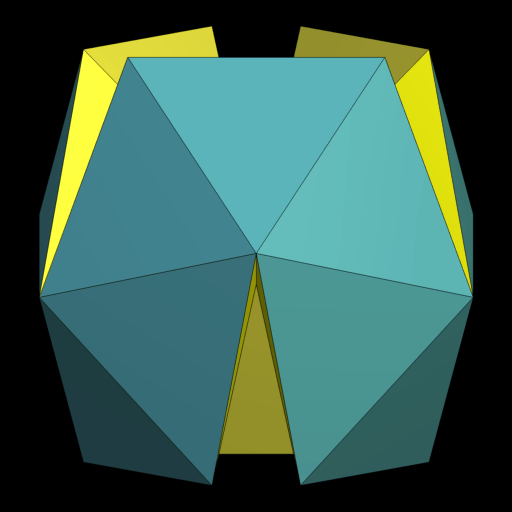### Deltahedra et al.

Convex polyhedra using only equilateral triangles for faces are called deltahedra. (Those are known such because of the shape of that upper-case Greek letter.) This kind of restriction could be considered a bit more generally, as well as for higher dimensions. The most general set-up there would be that of isohedral polytopes, being defined as having only a single shape for facets.

The list given here-below is not meant to be an exhaustive listing. It rather aims for some extremal ends: restricting to convex polytopes in general, and either to regular facets additionally or to non-isogonal results. – Note: this setup does not require the polytopes generally to be isogonal in addition, i.e. having just a single type of vertices (vertex surroundings / vertex figures). Polytopes which are both, isogonal and isohedral, commonly are known under the attribute noble (and thus are listed there).

`©`

As an aside it should be mentioned however, that already some results for non-convex deltahedra have been obtained. E.g. Rausenberger in 1915 and Cundy in 1952 were initiating a research which Olshevsky in 2006 brought to its avered end: the biform acoptic deltahedra (where acoptic refers to non-convex but still non-intersecting).

The right animated non-convex polyhedra then would be a triform acoptic deltahedron with 36 faces and a triform acoptic deltahedron with 120 faces respectively.

For all simplicial polytopes in general the set of Dehn-Summerville equations can be stated.

```----
2D
----
```
##### Facets being {4}
•   6 {4} → cube cube (regular)
##### Facets being {5}
• 12 {5} → doe dodecahedron (regular)

```----
3D
----
```
##### Facets being cubes
• 8 cubetes tesseract (regular)
##### Facets being octahedra
• 24 octico icositetrachoron (regular)
##### Facets being dodecahedra
• 120 doehi hecatonicosachoron (regular)

```----
4D
----
```
##### Facets being tesseracts
• 10 tespent penteract (regular)